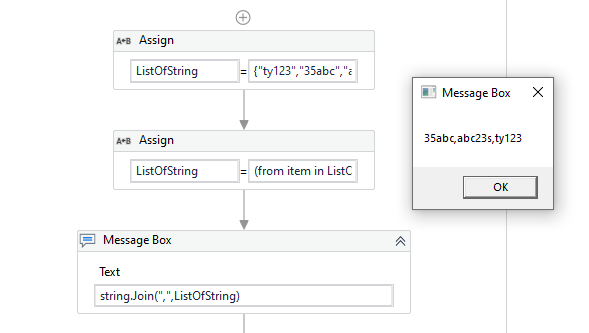# Sorting Alphanumeric strings

How can i sort alphanumeric strings in ascending order?

For eg: I have an array of strings like {34fghj4,asdg456,bhfg23s,bhfg12b,tyui123}
I want to sort it in ascending order like – {34fghj4,tyui123,bhfg12b,bhfg23s,asdg456}
where the string containing the numerical in the first place is considered the smallest)

Hi,

Can you elaborate the sorting rule?

Regards,

-Number always gets the priority. eg: In below
34fghj4
asdg456
bhfg23s
bhfg12b
tyui123

34fghj4 is taken first, then tyui123 followed by ,bhfg12b,bhfg23s

Hi,

Why tyui123 is smaller than bhfg12b? I suppose the former is 123 and the latter is 12?

Regards,

Numerical always gets the first priority, we need to select the string based on the postion of numberical. in tyui123 and bhfg12b – in the last position tyu i123 has 3 which is a numerical
.hence it gets priority

Hi @shreyaank,

Can you try the below expression

(From item in yourListOfString Order by item ascending select item)in your example, the order has to be 35abc,ty123,abc23s…because ty123 has 1 in the 3rd position and abc23s has c in the third position. hence, ty123 gets priority

can you share the datatype use for ListOfString ?

I have used it as Array of string

Hi,

Sorry but I couldn’t get your logic. Can you share step by step logic for your sorting?

First, evaluate numeric character position which appears first. So 34fghj4 is first position, is this right so far?
The first numeric character of all remaining is 5th. We need next evaluation for them.
Next, how do we evaluate these string?

Regards,

so, the logic is numerical takes priority. And the place at which numerical is placed takes priority and the lesser numerical value takes priority(ascending)

Hi,

If i understand your requirement correctly, the following will work.

``````arrStr = arrStr.OrderBy(Function(s) Int64.Parse(System.Text.RegularExpressions.Regex.Replace(s,"\D","A"), System.Globalization.NumberStyles.HexNumber)).ToArray
``````

Please note that this expression assumes length of each item is same.

Regards,

1 Like